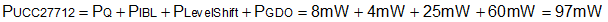SLUSCE9B June   2017  – March 2020

PRODUCTION DATA.

1. Features
2. Applications
3. Description
1.     Device Images
4. Revision History
5. Pin Configuration and Functions
6. Specifications
7. Detailed Description
1. 7.1 Overview
2. 7.2 Functional Block Diagram
3. 7.3 Feature Description
4. 7.4 Device Functional Modes
8. Application and Implementation
1. 8.1 Application Information
2. 8.2 Typical Application
9. Power Supply Recommendations
10. 10Layout
11. 11Device and Documentation Support
12. 12Mechanical, Packaging, and Orderable Information

• D|8

8.2.2.7 Estimate the UCC27712 Power Losses (PUCC27712)

The power losses of UCC27712 (PUCC27712) are estimated by calculating losses from several components. The gate drive loss in the UCC27712 is typically dominated by gate drive losses associated with charging and discharging the power device gate charge. There are other losses to consider especially if operating at high switching frequencies outlined below.

To determine the UCC27712 operating with no driver load, refer to the Typical Characteristics Figure 26 for IDD and IHB to determine the operating current at the appropriate fSW. The operating current power losses with no driver load are calculated in Equation 15:

Equation 15.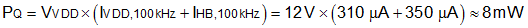Static losses due to leakage current (IBL) are calculated from the HB high-voltage node as shown in Equation 16:

Equation 16.Equation 17 calculates dynamic losses during the operation of the level shifter at HO turn-off edge. QP, typically 0.6 nC, is the charge absorbed by the level shifter during operation at each edge. Please note that if high-voltage switching occurs during HO turn-on as well (as in the case of ZVS topologies), then the power loss due to this component must be effectively doubled.

Equation 17.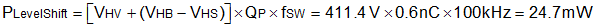where

• VHV: DC link high voltage input in V
• fSW: Switching frequency of converter in Hz.

Dynamic losses incurred due to the gate charge while driving the FETs Q1 and Q2 are calculated Equation 18. Please note that this component typically dominates over the dynamic losses related to the internal VDD and VHB switching logic circuitry in UCC27712. The losses incurred driving the gate charge are not all dissipated in the gate driver device, this includes losses in the external gate resistance and internal power switch gate resistance.

Equation 18.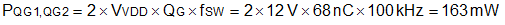The UCC27712 gate driver loss on the output stage ,PGDO, is part of PQG1,QG2. If the external gate resistances are zero most of the PQG1,QG2 will be dissipated in the UCC27712. If there are external gate resistances, the total loss will be distributed between the gate driver pull-up/down resistances and the external gate resistances.

The gate drive power dissipated within the UCC27712 driver can be determined by Equation 19:

Equation 19.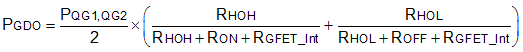In this example the gate drive related losses are approximately 60mW as shown in Equation 20:

Equation 20.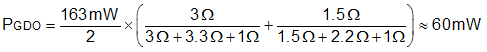For the conditions, VDD=12V, VHB = 400V, HO On-state Duty cycle D = 50%, QG = 68nC, fSW = 100kHz, the total power loss in UCC27712 driver for a half bridge power supply topology can be estimated as follows:

Equation 21.